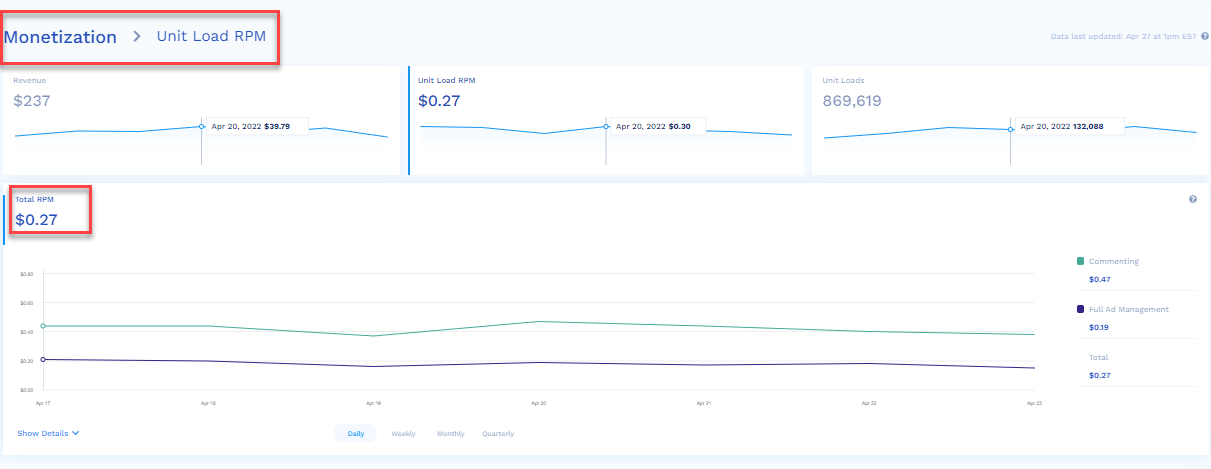Monetization provides 3 small-sized line graphs. These 3 line graphs are clickable.

The line graphs are for the following:

• Revenue

Unit Load RPM is the total revenue per thousand unit loads and is calculated by taking the total revenue for the selected time period, and dividing it by the total number of unit loads, then multiplying by 1,000.

When looking at RPM in a rolled-up view of Daily, Weekly, Monthly, or Quarterly, the calculation is the same, but the time frame of the data will be driven by each period within the roll-up.When you click the Unit Load RPM line graph, the total revenue for the selected date range is displayed as a line graph in detail. To view this page, you can also navigate from the left pane as Monetization > Unit Load RPM.

The features of the Unit Load RPM page are:

• The green section of the line graph is the total Unit Load RPM generated by the Commenting unit over the selected period of time (by default it is seven days and the maximum is 30 days).
• The blue section of the line graph is the total Unit Load RPM generated by the Full Ad Management unit over the selected period of time (by default it is seven days and the maximum is 30 days)

📘

Information

The image has 2 different products, Commenting and Trivia & Polls, hence red/blue is stacked on the graph. If you have only one Insticator product, you will just see one value in the table.

• Hover the mouse over the line graph to view the Unit Load RPM for that particular day.

• On the right side, you will see the following color-coded analytics for the selected period of time (by default it is seven days and the maximum is 30 days):
Total Unit Load RPM generated by the Commenting unit